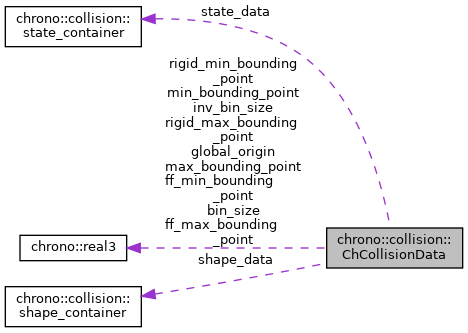chrono::collision::ChCollisionData Class Reference

Description

Global data for the custom Chrono multicore collision system.

#include <ChCollisionData.h>

Collaboration diagram for chrono::collision::ChCollisionData:[legend]

Public Member Functions

ChCollisionData (bool owns_data)

Public Attributes

bool owns_state_data
if false, state data set from outside

state_container state_data
state data arrays

shape_container shape_data
shape information data arrays

real collision_envelope
collision envelope for rigid shapes

real p_collision_envelope
collision envelope for 3-dof particles

short2 p_collision_family
collision family and family mask for 3-dof particles

std::vector< real3aabb_min
list of bounding boxes minimum point

std::vector< real3aabb_max
list of bounding boxes maximum point

std::vector< long long > pair_shapeIDs
shape IDs for each shape pair (encoded in a single long long)

std::vector< long long > contact_shapeIDs
shape IDs for each contact (encoded in a single long long)

std::vector< real3norm_rigid_rigid
[num_rigid_contacts] normal for each rigid-rigid contact

std::vector< real3cpta_rigid_rigid
[num_rigid_contacts] point on first shape for each rigid-rigid contact

std::vector< real3cptb_rigid_rigid
[num_rigid_contacts] point on second shape for each rigid-rigid contact

std::vector< real > dpth_rigid_rigid
[num_rigid_contacts] penetration depth for each rigid-rigid contact

[num_rigid_contacts] effective contact radius for each rigid-rigid contact

std::vector< vec2 > bids_rigid_rigid
[num_rigid_contacts] body IDs for each rigid-rigid contact pair

std::vector< real3norm_rigid_fluid
[num_rigid_fluid_contacts]

std::vector< real3cpta_rigid_fluid
[num_rigid_fluid_contacts]

std::vector< real > dpth_rigid_fluid
[num_rigid_fluid_contacts]

std::vector< int > neighbor_rigid_fluid
[num_rigid_fluid_contacts]

std::vector< int > c_counts_rigid_fluid
[num_fluid_bodies]

std::vector< int > neighbor_3dof_3dof
[num_fluid_contacts]

std::vector< int > c_counts_3dof_3dof
[num_fluid_contacts]

std::vector< int > particle_indices_3dof

std::vector< int > reverse_mapping_3dof

vec3 bins_per_axis
number of slices along each axis of the collision detection grid

real3 bin_size
bin sizes in each direction

real3 inv_bin_size
bin size reciprocals in each direction

real3 min_bounding_point
LBR (left-bottom-rear) corner of union of all AABBs.

real3 max_bounding_point
RTF (right-top-front) corner of union of all AABBs.

real3 global_origin
grid zero point (same as LBR)

uint num_bins
total number of bins

uint num_bin_aabb_intersections
number of bin - shape AABB intersections

uint num_active_bins
number of bins intersecting at least one shape AABB

uint num_possible_collisions
number of candidate collisions from broadphase

real3 rigid_min_bounding_point
LBR (left-bottom-rear) corner of union of rigid AABBs.

real3 rigid_max_bounding_point
RTF (right-top-front) corner of union of rigid AABBs.

vec3 ff_bins_per_axis
grid resolution for fluid particles

real3 ff_min_bounding_point
LBR (left-bottom-rear) corner of union of fluid AABBs.

real3 ff_max_bounding_point
RTF (right-top-front) corner of union of fluid AABBs.

std::vector< uint > bin_intersections
[num_rigid_shapes+1] number of bin intersections for each shape AABB

std::vector< uint > bin_number
[num_bin_aabb_intersections] bin index for bin-shape AABB intersections

std::vector< uint > bin_aabb_number
[num_bin_aabb_intersections] shape ID for bin-shape AABB intersections

std::vector< uint > bin_active
[num_active_bins] bin index of active bins (no duplicates)

std::vector< uint > bin_start_index
[num_active_bins+1]

std::vector< uint > bin_start_index_ext
[num_bins+1]

std::vector< uint > bin_num_contact
[num_active_bins+1]

uint num_rigid_shapes
number of collision models in a system

uint num_rigid_contacts
number of contacts between rigid bodies in a system

uint num_rigid_fluid_contacts
number of contacts between rigid and fluid objects

uint num_fluid_contacts
number of contacts between fluid objects

The documentation for this class was generated from the following file:
• /builds/uwsbel/chrono/src/chrono/collision/chrono/ChCollisionData.h